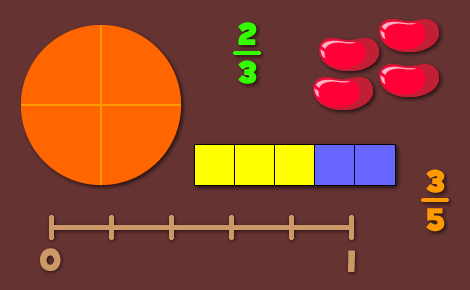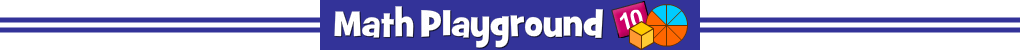Thinking Deeply with FractionsOne of the best ways to transform your math instruction and inspire mathematical thinking is to pose problems that encourage deep exploration. Open ended questions, such as those with multiple pathways and solutions can promote lively discussions and reveal your students’ thinking in unexpected ways. In this post, we’ll look at traditional tasks given to students who are learning fractions and simple ways to revise those tasks. This material is applicable to all grade levels, and especially well-suited for 3rd grade math.

###### Closed Question
Students are often asked to color one-fourth of a group. The purpose of this exercise is to determine if your students can identify a fraction of a set of objects. Students would typically count the number of objects and divide the set into four equal groups. Let’s see what happens when we make one small change to this task.

###### Open Question
Ms. Jackson has a bag of marbles. One-fourth of the marbles are blue. How many marbles are in the bag?

We’re still working with a fraction of a set but now we have an infinite number of solutions. Students can make the total any number they wish provided it’s a multiple of 4. Ask your students why that must always be true.
###### Closed Question
Shade a rectangle to show the fraction, 3/5.

In this problem, the rectangle is usually divided into 5 equal parts at the start. Students only need to recognize that the numerator, 3, tells the number of parts to shade.

###### Open Question
How many different ways can you show the fraction, 3/5?

Students may still use a rectangle to represent 1 whole but now they are free to divide the rectangle in a variety of ways. Even better, students are no longer limited to a rectangular model. They can use other shapes, a set of objects, or a number line.
###### Closed Question
Find the sum of 2/3 and 2/6.

This task has only one right answer although students may be creative in the process of adding fractions.

###### Open Question
Find two fractions that add to 1.
Find three fractions that add to 1.

Now the task has an endless number of solutions. These solutions can provide a lot of information about your students’ facility with fractions. One student may add two halves to find one whole. Another may use a unit fraction and its complement such as 1/4 + 3/4 or 1/100 + 99/100.
###### Closed Question
Place the following fractions on a number line.
3/5, 4/5, and 7/10

###### Open Question
3/5 and 4/5 are placed on a number line. What fraction(s) could you place between them?
###### Closed Question
Show 2/3 of a given shape.

###### Open Question
The given shape is 2/3 of another shape. Can you draw the whole shape?
###### Closed Question
Use the symbols <, > or = to compare the following fractions.
1/4 and 1/3
2/3 and 6/9
3/4 and 2/5

###### Open Question
Find 3 fractions smaller than 1/3.
Find 3 fractions equal to 2/3.
Find 3 fractions greater than 2/5.
Effective tasks are ones that encourage thinking and analysis, enable students to build upon previous knowledge, and reveal misunderstandings. Open ended tasks are naturally, and more accurately, differentiating because they encourage creativity. They provide a window into each student’s thought process, making them very useful assessment tools.